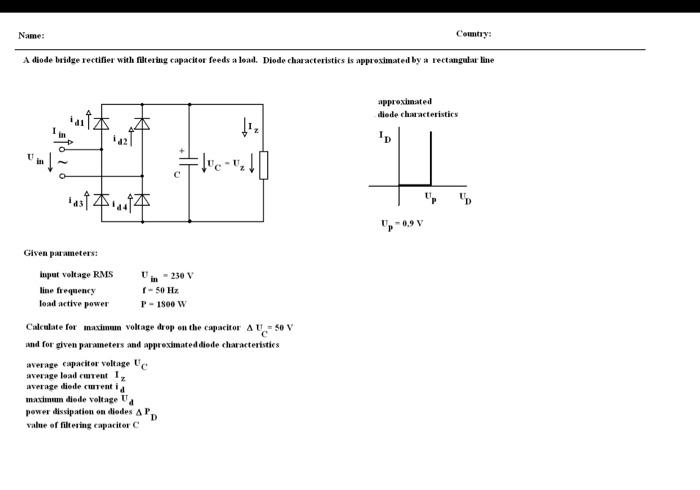# Question Solved1 AnswerName: Country: A diode beilge rectifier with filtering capacitor feeds a lead. Diode characteristics is approximatel by a rectangulat line approximated diede chacteristics . lue- c tusf本.. Given preters: Input voltage RMS - 230 V line frequency 1-50 Hz lead active power P-1800 W Calculate for maximum voltage drop on the capacitor ay = 50 V and for given parameters and approximated diode characteristics average capacitor voltage U average load current 1, average diode curentia maximun diede voltage U power dissipation on diedes AP value of filtering capacitor C DWRK369 The Asker · Electrical EngineeringTranscribed Image Text: Name: Country: A diode beilge rectifier with filtering capacitor feeds a lead. Diode characteristics is approximatel by a rectangulat line approximated diede chacteristics . lue- c tusf本.. Given preters: Input voltage RMS - 230 V line frequency 1-50 Hz lead active power P-1800 W Calculate for maximum voltage drop on the capacitor ay = 50 V and for given parameters and approximated diode characteristics average capacitor voltage U average load current 1, average diode curentia maximun diede voltage U power dissipation on diedes AP value of filtering capacitor C D
More
Transcribed Image Text: Name: Country: A diode beilge rectifier with filtering capacitor feeds a lead. Diode characteristics is approximatel by a rectangulat line approximated diede chacteristics . lue- c tusf本.. Given preters: Input voltage RMS - 230 V line frequency 1-50 Hz lead active power P-1800 W Calculate for maximum voltage drop on the capacitor ay = 50 V and for given parameters and approximated diode characteristics average capacitor voltage U average load current 1, average diode curentia maximun diede voltage U power dissipation on diedes AP value of filtering capacitor C D